## Galerkin BEM for 3D Laplace Equation

Download GBEM_LAP, a package for solving the 3D Laplace equation based on a piecewise linear Galerkin Boundary Element Method.

# Piecewise linear Galerkin method

The potential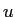and flux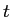are assumed to be linear over each boundary element. It can be shown (see [2,3,4]) that the Galerkin surface integrals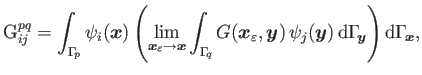and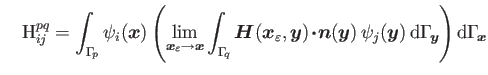can be employed to successfully computeandon the boundary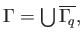where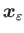is an exterior point to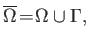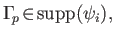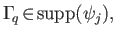and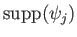stands for the support of the function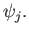Moreover,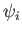is a linear test function defined over the flat triangle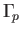and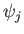is a linear shape function defined over the flat triangle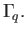In addition, the following potentials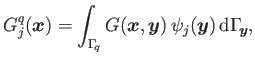and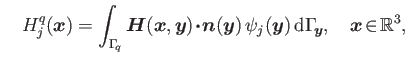are utilized to effectively calculateat interior points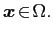The analytic expressions for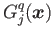and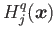over a flat triangle are given in .

# References

 S. Nintcheu Fata.
Explicit expressions for 3D boundary integrals in potential theory.
Int. J. Num. Meth. Eng., 78(1):32-47, 2009.
 S. Nintcheu Fata and L. J. Gray.
Semi-analytic integration of hypersingular Galerkin BIEs for three-dimensional potential problems.
J. Comput. Appl. Math., 231(2):561-576, 2009.
 S. Nintcheu Fata and L. J. Gray.
On the implementation of 3D Galerkin boundary integral equations.
Eng. Anal. Boundary Elem., 34(1):60-65, 2010.
 S. Nintcheu Fata.
Semi-analytic treatment of nearly-singular Galerkin surface integrals.
Appl. Numer. Math., 60(10):974-993, 2010.